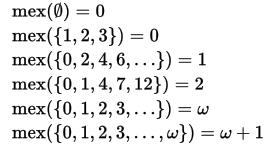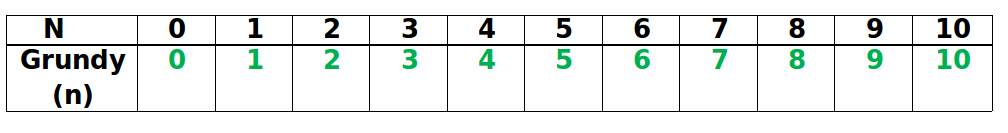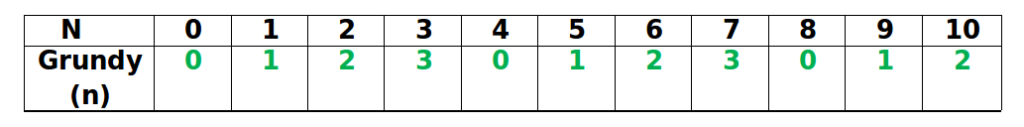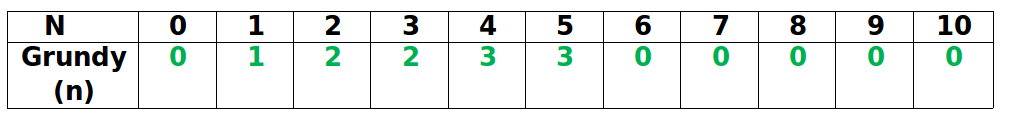# Combinatorial Game Theory | Set 3 (Grundy Numbers/Nimbers and Mex)

We have introduced Combinatorial Game Theory in Set 1 and discussed Game of Nim in Set 2.

Grundy Number is a number that defines a state of a game. We can define any impartial game (example : nim game) in terms of Grundy Number.

Grundy Numbers or Nimbers determine how any Impartial Game (not only the Game of Nim) can be solved once we have calculated the Grundy Numbers associated with that game using Sprague-Grundy Theorem.

But before calculating Grundy Numbers, we need to learn about another term- Mex.

What is Mex?
‘Minimum excludant’ a.k.a ‘Mex’ of a set of numbers is the smallest non-negative number not present in the set.How to calculate Grundy Numbers?
We use this definition- The Grundy Number/ nimber is equal to 0 for a game that is lost immediately by the first player and is equal to Mex of the nimbers of all possible next positions for any other game.

Below are three example games and programs to calculate Grundy Number and Mex for each of them. Calculation of Grundy Numbers is done basically by a recursive function called as calculateGrundy() function which uses calculateMex() function as its sub-routine.

Example 1
The game starts with a pile of n stones, and the player to move may take any positive number of stones. Calculate the Grundy Numbers for this game. The last player to move wins. Which player wins the game?

Since if the first player has 0 stones, he will lose immediately, so Grundy(0) = 0

If a player has 1 stones, then he can take all the stones and win. So the next possible position of the game (for the other player) is (0) stones

Hence, Grundy(1) = Mex(0) = 1 [According to the definition of Mex]

Similarly, If a player has 2 stones, then he can take only 1 stone or he can take all the stones and wins. So the next possible position of the game (for the other player) is (1, 0) stones respectively.

Hence, Grundy(2) = Mex(0, 1) = 2 [According to the definition of Mex]

Similarly, If a player has ‘n’ stones, then he can take only 1 stone, or he can take 2 stones…….. or he can take all the stones and win. So the next possible position of the game (for the other player) is (n-1, n-2,….1) stones respectively.

Hence, Grundy(n) = Mex (0, 1, 2, ….n-1) = n [According to the definition of Mex]
We summarize the first the Grundy Value from 0 to 10 in the below table-## C++

 `/* A recursive C++ program to find Grundy Number for ` `   ``a game which is like a one-pile version of Nim. ` `  ``Game Description : The game starts with a pile of n stones, ` `  ``and the player to move may take any positive number of stones.  ` `The last player to move wins. Which player wins the game? */` `#include ` `using` `namespace` `std; ` ` `  `// A Function to calculate Mex of all the values in ` `// that set. ` `int` `calculateMex(unordered_set<``int``> Set) ` `{ ` `    ``int` `Mex = 0; ` ` `  `    ``while` `(Set.find(Mex) != Set.end()) ` `        ``Mex++; ` ` `  `    ``return` `(Mex); ` `} ` ` `  `// A function to Compute Grundy Number of 'n' ` `// Only this function varies according to the game ` `int` `calculateGrundy(``int` `n) ` `{ ` `    ``if` `(n == 0) ` `        ``return` `(0); ` ` `  `    ``unordered_set<``int``> Set; ``// A Hash Table ` ` `  `    ``for` `(``int` `i=0; i<=n-1; i++) ` `            ``Set.insert(calculateGrundy(i)); ` ` `  `    ``return` `(calculateMex(Set)); ` `} ` ` `  `// Driver program to test above functions ` `int` `main() ` `{ ` `    ``int` `n = 10; ` `    ``printf``(``"%d"``, calculateGrundy(n)); ` `    ``return` `(0); ` `} `

## Python3

 `''' A recursive Python3 program to find Grundy Number for ` `a game which is like a one-pile version of Nim. ` `Game Description : The game starts with a pile of n stones, ` `and the player to move may take any positive number of stones.  ` `The last player to move wins. Which player wins the game? '''` ` `  `# A Function to calculate Mex of all the values in ` `# that set. ` `def` `calculateMex(``Set``): ` `    ``Mex ``=` `0` ` `  `    ``while` `(Mex ``in` `Set``): ` `        ``Mex ``+``=` `1` ` `  `    ``return` `(Mex) ` ` `  `# A function to Compute Grundy Number of 'n' ` `# Only this function varies according to the game ` `def` `calculateGrundy( n): ` `    ``if` `(n ``=``=` `0``): ` `        ``return` `(``0``) ` ` `  `    ``Set` `=` `set``() ``# A Hash Table ` ` `  `    ``for` `i ``in` `range``(n): ` `        ``Set``.add(calculateGrundy(i)); ` ` `  `    ``return` `(calculateMex(``Set``)) ` ` `  `# Driver program to test above functions ` `n ``=` `10``; ` `print``(calculateGrundy(n)) ` ` `  `# This code is contributed by ANKITKUMAR34 `

Output :

`10`

The above solution can be optimized using Dynamic Programming as there are overlapping subproblems. The Dynamic programming based implementation can be found here.

Example 2
The game starts with a pile of n stones, and the player to move may take any positive number of stones up to 3 only. The last player to move wins. Which player wins the game? This game is 1 pile version of Nim.

Since if the first player has 0 stones, he will lose immediately, so Grundy(0) = 0

If a player has 1 stones, then he can take all the stones and win. So the next possible position of the game (for the other player) is (0) stones

Hence, Grundy(1) = Mex(0) = 1 [According to the definition of Mex]

Similarly, if a player has 2 stones, then he can take only 1 stone or he can take 2 stones and win. So the next possible position of the game (for the other player) is (1, 0) stones respectively.

Hence, Grundy(2) = Mex(0, 1) = 2 [According to the definition of Mex]

Similarly, Grundy(3) = Mex(0, 1, 2) = 3 [According to the definition of Mex]

But what about 4 stones ?
If a player has 4 stones, then he can take 1 stone or he can take 2 stones or 3 stones, but he can’t take 4 stones (see the constraints of the game). So the next possible position of the game (for the other player) is (3, 2, 1) stones respectively.

Hence, Grundy(4) = Mex (1, 2, 3) = 0 [According to the definition of Mex]

So we can define Grundy Number of any n >= 4 recursively as-

Grundy(n) = Mex[Grundy (n-1), Grundy (n-2), Grundy (n-3)]

We summarize the first the Grundy Value from 0 to 10 in the below table-## C++

 `/* A recursive C++ program to find Grundy Number for ` `a game which is one-pile version of Nim. ` `Game Description : The game starts with a pile of ` `n stones, and the player to move may take any ` `positive number of stones up to 3 only. ` `The last player to move wins. */` `#include ` `using` `namespace` `std; ` ` `  `// A Function to calculate Mex of all the values in ` `// that set. ` ` `  `// A function to Compute Grundy Number of 'n' ` `// Only this function varies according to the game ` `int` `calculateGrundy(``int` `n) ` `{ ` `    ``if` `(n == 0) ` `        ``return` `(0); ` `    ``if` `(n == 1) ` `        ``return` `(1); ` `    ``if` `(n == 2) ` `        ``return` `(2); ` `    ``if` `(n == 3) ` `        ``return` `(3); ` `    ``else` `        ``return` `(n%(3+1)); ` `} ` ` `  `// Driver program to test above functions ` `int` `main() ` `{ ` `    ``int` `n = 10; ` `    ``printf``(``"%d"``, calculateGrundy(n)); ` `    ``return` `(0); ` `} `

## Java

 `/* A recursive Java program to find  ` `Grundy Number for a game which is  ` `one-pile version of Nim. ` `Game Description : The game starts with ` `a pile of n stones, and the player to ` `move may take any positive number of stones  ` `up to 3 only.The last player to move wins. */` `import` `java.util.*; ` ` `  `class` `GFG ` `{ ` ` `  `     `  `    ``// A function to Compute Grundy  ` `    ``// Number of 'n' Only this function  ` `    ``// varies according to the game ` `    ``static` `int` `calculateGrundy(``int` `n)  ` `    ``{ ` `        ``if` `(n == ``0``)  ` `            ``return` `0``; ` `        ``if` `(n == ``1``)  ` `            ``return` `1``; ` `        ``if` `(n == ``2``)  ` `            ``return` `2``; ` `        ``if` `(n == ``3``) ` `            ``return` `3``; ` `        ``else` `            ``return` `(n%(``3``+``1``)); ` `    ``} ` ` `  `    ``// Driver code ` `    ``public` `static` `void` `main(String[] args) ` `    ``{ ` `        ``int` `n = ``10``; ` `        ``System.out.printf(``"%d"``, calculateGrundy(n)); ` `    ``} ` `}  ` `// This code is contributed by rahulnamdevrn27 `

## Python3

 `# A recursive Python3 program to find Grundy Number  ` `# for a game which is one-pile version of Nim.  ` `# Game Description : The game starts with a pile  ` `# of n stones, and the player to move may take  ` `# any positive number of stones up to 3 only.  ` `# The last player to move wins. ` ` `  ` `  `  `  `# A function to Compute Grundy Number of 'n'  ` `# Only this function varies according to the game  ` `def` `calculateGrundy(n):  ` ` `  `    ``if` `0` `<``=` `n <``=` `3``: ` `        ``return` `n ` `     `  `    ``else``: ` `        ``return` `(n``%``(``3``+``1``)); ` `       `  `    `  `  `  `# Driver program to test above functions  ` `if` `__name__ ``=``=` `"__main__"``:  ` `  `  `    ``n ``=` `10`  `    ``print``(calculateGrundy(n))  ` `     `  `# This code is contributed by rahulnamdevrn27 `

## C#

 `/* A recursive Java program to find Grundy Number  ` `for a game which is one-pile version of Nim.  ` `Game Description : The game starts with a pile of  ` `n stones, and the player to move may take any  ` `positive number of stones up to 3 only.The last  ` `player to move wins. */` `using` `System;  ` `using` `System.Collections.Generic; ` ` `  `class` `GFG  ` `{  ` ` `  `     `  `    ``// A function to Compute Grundy Number of  ` `    ``// 'n' Only this function varies according  ` `    ``// to the game  ` `    ``static` `int` `calculateGrundy(``int` `n)  ` `    ``{  ` `        ``if` `(n == 0)  ` `            ``return` `0; ` `        ``if` `(n == 1)  ` `            ``return` `1; ` `        ``if` `(n == 2)  ` `            ``return` `2; ` `        ``if` `(n == 3) ` `            ``return` `3; ` `        ``else` `            ``return` `(n%(3+1)); ` `         `  `    ``}  ` ` `  `    ``// Driver code  ` `    ``public` `static` `void` `Main(String[] args)  ` `    ``{  ` `        ``int` `n = 10;  ` `        ``Console.Write(calculateGrundy(n));  ` `    ``}  ` `}  ` `// This code is contributed by rahulnamdevrn27 `

Output :

`2`

The general solution for above code when we are allowed to pick upto k stones can be found here.

Example 3
The game starts with a number- ‘n’ and the player to move divides the number- ‘n’ with 2, 3 or 6 and then takes the floor. If the integer becomes 0, it is removed. The last player to move wins. Which player wins the game?

We summarize the first the Grundy Value from 0 to 10 in the below table:Think about how we generated this table.

## C++

 `/* A recursive C++ program to find Grundy Number for ` `   ``a game. ` ` ``Game Description:  The game starts with a number- 'n' ` ` ``and the player to move divides the number- 'n' with 2, 3 ` ` ``or 6 and then takes the floor. If the integer becomes 0, ` ` ``it is removed. The last player to move wins.  */` `#include ` `using` `namespace` `std; ` ` `  `// A Function to calculate Mex of all the values in ` `// that set. ` `int` `calculateMex(unordered_set<``int``> Set) ` `{ ` `    ``int` `Mex = 0; ` ` `  `    ``while` `(Set.find(Mex) != Set.end()) ` `        ``Mex++; ` ` `  `    ``return` `(Mex); ` `} ` ` `  `// A function to Compute Grundy Number of 'n' ` `// Only this function varies according to the game ` `int` `calculateGrundy (``int` `n) ` `{ ` `    ``if` `(n == 0) ` `        ``return` `(0); ` ` `  `    ``unordered_set<``int``> Set; ``// A Hash Table ` ` `  `    ``Set.insert(calculateGrundy(n/2)); ` `    ``Set.insert(calculateGrundy(n/3)); ` `    ``Set.insert(calculateGrundy(n/6)); ` ` `  `    ``return` `(calculateMex(Set)); ` `} ` ` `  `// Driver program to test above functions ` `int` `main() ` `{ ` `    ``int` `n = 10; ` `    ``printf``(``"%d"``, calculateGrundy (n)); ` `    ``return` `(0); ` `} `

## Java

 `/* A recursive Java program to find Grundy Number for ` `a game. ` `Game Description : The game starts with a number- 'n' ` `and the player to move divides the number- 'n' with 2, 3 ` `or 6 and then takes the floor. If the integer becomes 0, ` `it is removed. The last player to move wins. */` `import` `java.util.*; ` ` `  `class` `GFG  ` `{ ` ` `  `    ``// A Function to calculate Mex of all the values in ` `    ``// that set. ` `    ``static` `int` `calculateMex(HashSet Set)  ` `    ``{ ` `        ``int` `Mex = ``0``; ` ` `  `        ``while` `(Set.contains(Mex))  ` `        ``{ ` `            ``Mex++; ` `        ``} ` ` `  `        ``return` `(Mex); ` `    ``} ` ` `  `    ``// A function to Compute Grundy Number of 'n' ` `    ``// Only this function varies according to the game ` `    ``static` `int` `calculateGrundy(``int` `n)  ` `    ``{ ` `        ``if` `(n == ``0``)  ` `        ``{ ` `            ``return` `(``0``); ` `        ``} ` ` `  `        ``HashSet Set = ``new` `HashSet(); ``// A Hash Table ` ` `  `        ``Set.add(calculateGrundy(n / ``2``)); ` `        ``Set.add(calculateGrundy(n / ``3``)); ` `        ``Set.add(calculateGrundy(n / ``6``)); ` ` `  `        ``return` `(calculateMex(Set)); ` `    ``} ` ` `  `    ``// Driver code ` `    ``public` `static` `void` `main(String[] args)  ` `    ``{ ` `        ``int` `n = ``10``; ` `        ``System.out.printf(``"%d"``, calculateGrundy(n)); ` `    ``} ` `}  ` ` `  `// This code is contributed by PrinciRaj1992 `

## Python3

 `# A recursive Python3 program to  ` `# find Grundy Number for a game.  ` `# Game Description : The game starts with a number- 'n'  ` `# and the player to move divides the number- 'n' with 2, 3  ` `# or 6 and then take the floor. If the integer becomes 0,  ` `# it is removed. The last player to move wins. ` ` `  `# A Function to calculate Mex  ` `# of all the values in that set.  ` `def` `calculateMex(``Set``):  ` `  `  `    ``Mex ``=` `0`  `    ``while` `Mex ``in` `Set``:  ` `        ``Mex ``+``=` `1` ` `  `    ``return` `Mex  ` `  `  `# A function to Compute Grundy Number of 'n'  ` `# Only this function varies according to the game  ` `def` `calculateGrundy(n):  ` `  `  `    ``if` `n ``=``=` `0``: ` `        ``return` `0`  ` `  `    ``Set` `=` `set``() ``# A Hash Table  ` ` `  `    ``Set``.add(calculateGrundy(n ``/``/` `2``))  ` `    ``Set``.add(calculateGrundy(n ``/``/` `3``))  ` `    ``Set``.add(calculateGrundy(n ``/``/` `6``))  ` ` `  `    ``return` `(calculateMex(``Set``))  ` `  `  `# Driver program to test above functions  ` `if` `__name__ ``=``=` `"__main__"``:  ` `  `  `    ``n ``=` `10`  `    ``print``(calculateGrundy(n))  ` `     `  `# This code is contributed by Rituraj Jain `

## C#

 `/* A recursive C# program to find Grundy Number for  ` `a game.  ` `Game Description: The game starts with a number- 'n'  ` `and the player to move divides the number- 'n' with 2, 3  ` `or 6 and then takes the floor. If the integer becomes 0,  ` `it is removed. The last player to move wins. */` `using` `System; ` `using` `System.Collections.Generic; ` ` `  `class` `GFG  ` `{  ` ` `  `    ``// A Function to calculate Mex of   ` `    ``// all the values in that set.  ` `    ``static` `int` `calculateMex(HashSet<``int``> Set)  ` `    ``{  ` `        ``int` `Mex = 0;  ` ` `  `        ``while` `(Set.Contains(Mex))  ` `        ``{  ` `            ``Mex++;  ` `        ``}  ` ` `  `        ``return` `(Mex);  ` `    ``}  ` ` `  `    ``// A function to Compute Grundy Number of 'n'  ` `    ``// Only this function varies according to the game  ` `    ``static` `int` `calculateGrundy(``int` `n)  ` `    ``{  ` `        ``if` `(n == 0)  ` `        ``{  ` `            ``return` `(0);  ` `        ``}  ` ` `  `        ``// A Hash Table  ` `        ``HashSet<``int``> Set = ``new` `HashSet<``int``>();  ` ` `  `        ``Set.Add(calculateGrundy(n / 2));  ` `        ``Set.Add(calculateGrundy(n / 3));  ` `        ``Set.Add(calculateGrundy(n / 6));  ` ` `  `        ``return` `(calculateMex(Set));  ` `    ``}  ` ` `  `    ``// Driver code  ` `    ``public` `static` `void` `Main()  ` `    ``{  ` `        ``int` `n = 10;  ` `        ``Console.WriteLine(calculateGrundy(n));  ` `    ``}  ` `}  ` ` `  `// This code is contributed by PrinciRaj1992 `

Output :

`0`

The above solution can be optimized using Dynamic Programming as there are overlapping subproblems. The Dynamic programming based implementation can be found here.

In the next post, we will be discussing solutions to Impartial Games using Grundy Numbers or Nimbers.

This article is contributed by Rachit Belwariar. If you like GeeksforGeeks and would like to contribute, you can also write an article and mail your article to contribute@geeksforgeeks.org. See your article appearing on the GeeksforGeeks main page and help other Geeks.

Attention reader! Don’t stop learning now. Get hold of all the important DSA concepts with the DSA Self Paced Course at a student-friendly price and become industry ready.

My Personal Notes arrow_drop_up

Article Tags :
Practice Tags :

6

Please write to us at contribute@geeksforgeeks.org to report any issue with the above content.